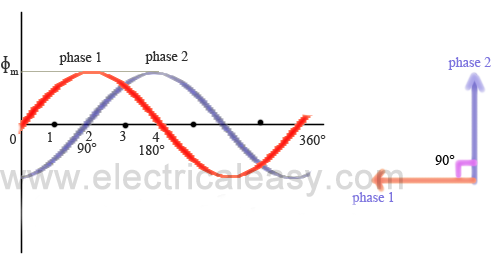# Production of rotating magnetic field in polyphase stator

In an induction motor, when AC supply is given to the the stator, magnetic flux is produced which is revolving at synchronous speed. This post will explain you in brief about production of rotating magnetic flux for 2 phase as well as 3 phase supply.

#### For 2 phase supply:fig (a)
Let the stator is wound for 2 phase supply. The two phases are kept 90 space degrees apart as illustrated in fig (a).

Let, Φ1 and  Φ2 be the instantaneous values of the fluxes set up by phase 1 and phase 2 respectively.

(i) When θ = 0° (at origin fig. a), magnitude of the flux set up by phase-1 will be 0 and the magnitude of the flux by phase 2 will be maximum but in negative direction. This is illustrated in fig (b). Hence the magnitude of the resultant flux Φr will be equal to Φm.

(ii) θ = 45° (position 1 in fig a)
Flux by phase-1 >> Φ1 = sqrt.2 * Φm.
Flux by phase-2  >> Φ2 = sqrt.2 * Φm.
Hence resultant flux >> Φr = Φm.
But the resultant has shifted 45 degrees clockwise.

(iii) θ = 90° (position 2)
Flux by phase-1 >> Φ1 = Φm.
Flux by phase-2  >> Φ2 = 0.
Hence resultant flux >> Φr = Φm.
But the resultant has further shifted 45 degrees clockwise OR resultant has shifted 90 degrees from its initial position.

(iv) θ = 135° (position 3)
Flux by phase-1 >> Φ1 = Φm.
Flux by phase-2  >> Φ2 = Φm.
Hence resultant flux >> Φr = Φm.
But the resultant has further shifted 45 degrees clockwise OR resultant has shifted 135 degrees from its initial position.

(iv) θ = 180° (position 4)
Flux by phase-1 >> Φ1 = 0.
Flux by phase-2  >> Φ2 = Φm.
Hence resultant flux >> Φr = Φm.
But the resultant has further shifted 45 degrees clockwise OR resultant has shifted 180 degrees from its initial position.
Thus, it can be concluded that the magnitude of the resultant flux remains constant but its direction keeps rotating clockwise.

The speed of the rotating magnetic flux is called as synchronous speed (Ns) and it is given by
where, f =frequency of the supply and  P = number of poles.

#### 3 Phase supply:

Similarly, for a three phase supply, following figures will illustrate.